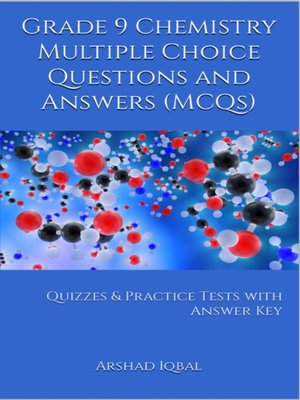## ebook ∣ Quizzes & Practice Tests with Answer Key (Chemistry Quick Study Guides & Terminology Notes about Everything) · Chemistry Quick Study Guides & Terminology Notes about EverythingWith an OverDrive account, you can save your favorite libraries for at-a-glance information about availability. Find out more about OverDrive accounts.

### Title found at these libraries:

Grade 9 Chemistry Multiple Choice Questions and Answers (MCQs) PDF: Quiz & Practice Tests with Answer Key (9th Grade Chemistry Quick Study Guide & Terminology Notes about Everything) includes revision guide for problem solving with 250 solved MCQs. Grade 9 Chemistry MCQ with answers PDF book covers basic concepts, theory and analytical assessment tests. "Grade 9 Chemistry MCQ" book PDF helps to practice test questions from exam prep notes.

Grade 9 chemistry quick study guide provides 250 verbal, quantitative, and analytical reasoning past question papers, solved MCQs. Grade 9 Chemistry Multiple Choice Questions and Answers (MCQs) PDF download, a book to practice quiz questions and answers on chapters: Chemical reactivity, electrochemistry, fundamentals of chemistry, periodic table and periodicity, physical states of matter, solutions, structure of atoms, structure of molecules tests for school and college revision guide. Grade 9 Chemistry Quiz Questions and Answers PDF book covers beginner's questions, textbook's study notes to practice tests.

Chemistry practice tests with answer key includes high school worksheets for self-assessment competitive exams. Grade 9 chemistry MCQ book, a quick study guide for NEET/MCAT/GRE/GMAT/SAT/ACT certification exam questions. "9th Grade Chemistry MCQs" PDF book covers problem solving tests from chemistry textbook's chapters as:

Chapter 1: Chemical Reactivity MCQs
Chapter 2: Electrochemistry MCQs
Chapter 3: Fundamentals of Chemistry MCQs
Chapter 4: Periodic Table and Periodicity MCQs
Chapter 5: Physical States of Matter MCQs
Chapter 6: Solutions MCQs
Chapter 7: Structure of Atoms MCQs
Chapter 8: Structure of Molecules MCQs

Solve "Chemical Reactivity MCQ" book PDF, chapter 1 to solve MCQ questions and answers: Metals, and non-metals.

Solve "Electrochemistry MCQ" book PDF, chapter 2 to solve MCQ questions and answers: Corrosion and prevention, electrochemical cells, electrochemical industries, oxidation and reduction, oxidation reduction and reactions, oxidation states, oxidizing and reducing agents.

Solve "Fundamentals of Chemistry MCQ" book PDF, chapter 3 to solve MCQ questions and answers: Atomic and mass number, Avogadro number and mole, branches of chemistry, chemical calculations, elements and compounds particles, elements compounds and mixtures, empirical and molecular formulas, gram atomic mass molecular mass and gram formula, ions and free radicals, molecular and formula mass, relative atomic mass, and mass unit.

Solve "Periodic Table and Periodicity MCQ" book PDF, chapter 4 to solve MCQ questions and answers: Periodic table, periodicity and properties.

Solve "Physical States of Matter MCQ" book PDF, chapter 5 to solve MCQ questions and answers: Allotropes, gas laws, liquid state and properties, physical states of matter, solid state and properties, types of bonds, and typical properties.

Solve "Solutions MCQ" book PDF, chapter 6 to solve MCQ questions and answers: Aqueous solution solute and solvent, concentration units, saturated unsaturated supersaturated and dilution of solution, solubility, solutions suspension and colloids, and types of solutions.

Solve "Structure of Atoms MCQ" book PDF, chapter 7 to solve MCQ questions and answers: Atomic structure experiments, electronic configuration, and isotopes.

Solve "Structure of Molecules MCQ" book PDF, chapter 8 to solve MCQ questions and answers: Atoms reaction, bonding nature and properties, chemical bonds, intermolecular forces, and types of bonds.